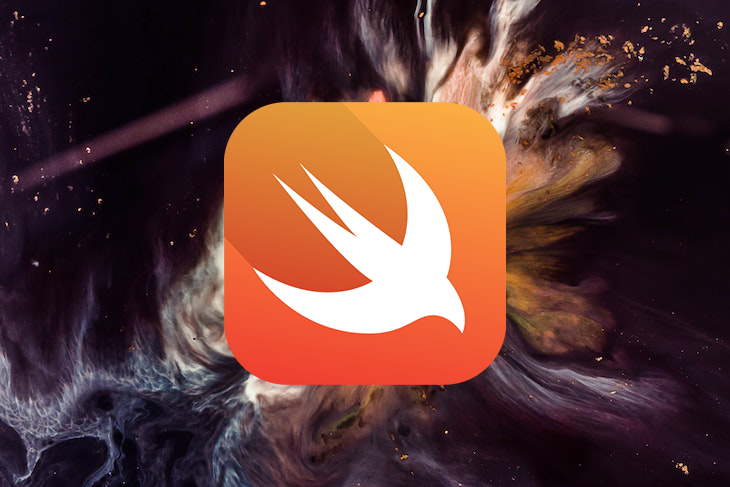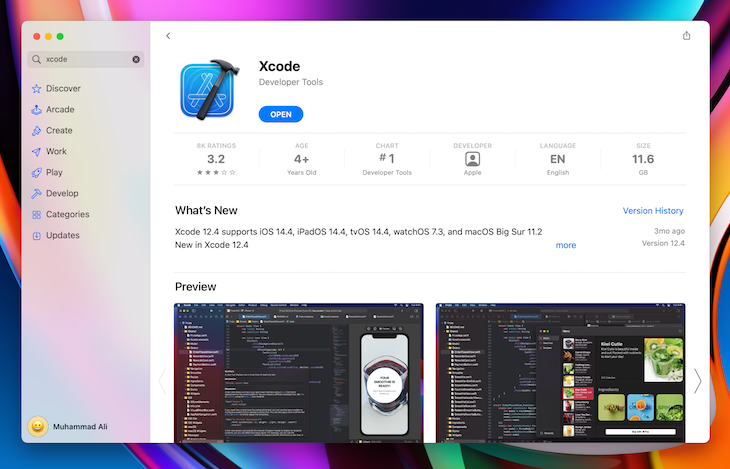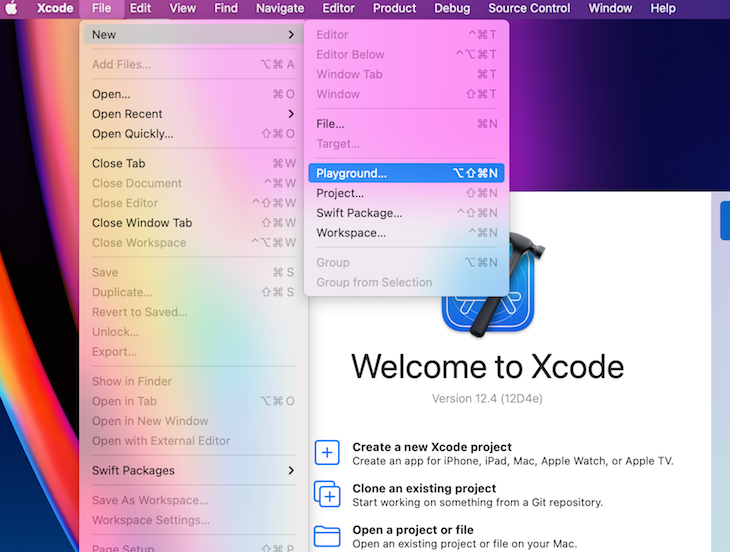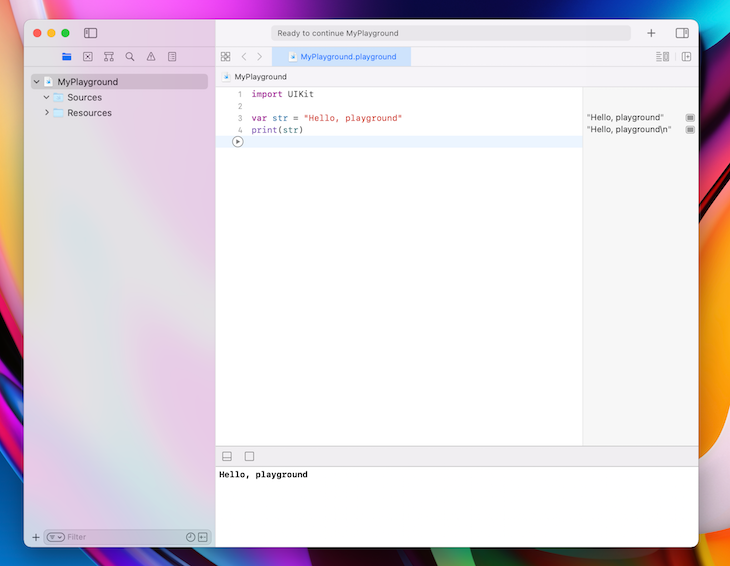# Mastering Swift fundamentalsSwift, a programming language released by Apple in 2014, has quickly become one of the fastest growing languages in history. If you are interested in building apps for macOS or iOS, Swift is the best language choice in my opinion.

In this tutorial, we’ll become familiar with Swift by learning its basic operations and practicing with some relevant examples. Let’s get started!

## Installation

Swift is available on Mac, Windows, and Linux in version 5.4.1. Installing Swift is easy using Xcode, Apple’s free workflow for desktop and native iOS development.You can download Xcode either from the App Store inside macOS or on the official Swift website. If you’re using Windows or Linux, download and install Swift from its official site.

If you don’t want to install Swift on your computer, you can try an online Playground for Swift.

### Open an Xcode Playground

Once you’ve installed Swift on your computer, open a Playground using Xcode, where you can begin writing your code.## Data types

We need to know what data types are supported in Swift to understand what we can accomplish. We’ll use the following six data types:

Data type Example Represents Purpose
Character `s`, `w` A 16-bit Unicode character Single character editing
String `swift language` Text data Saving, updating, modifying a text/message
Int `5`, `-5` An integer number Represents any integer number
Float `5.5`, `-5.5` 32-bit floating-point number Represents a 32-bit floating-point number
Double `5.5`, `-5.5` 64-bit floating-point number Represents a 64-bit floating-point number
Bool `true`, `false` Represent states (true/false) Provides true/false value to use in our program

## Variables

Writing a program requires a lot of data. Variables provide a way to label data, making it easier to modify and reference.

### Variable structure

We’ll use the following structure to format a variable: `Keyword variableName:dataType`. You can see the structure used in the code block below:

```var name:String
```

When we define a variable using the structure above, we don’t put any data in the variable initially. Let’s add data in the variable to modify it from a `null`, which represents an empty value in programming, to a `string`:

```var name:String = "nerdjfpb"
```

Later, we can reference the code above as needed to access the data. For example, `print(name)` will show `nerdjfpb` on the Playground console. You can think of printing something as displaying the result. We can see what value is inside the name.

We can change the variable value easily:

```name = "Nerd Jfpb"
```

When writing in Swift, we don’t need to specify the data type when we begin with its value. For example, in the following code, Swift understands the name is a string:

```var name = "nerdjfpb"
```

If we write the code block below, we’ll receive an error. Because Swift is a typed language, we can’t change the data type of a variable:

```name = 5
```

Imagine that you want to reference a variable through your programming but don’t want to change its value. Swift provides a specific method for referencing variables.

### Referencing variables

To reference a variable, we’ll use the following structure: `Keyword(let) variableName dataType = Value`, which can be seen in the example below:

``````let ownerName = "Nerdjfpb"
``````

We can only define the value of `ownerName` once, and we can’t change it. The value will remain constant throughout the entire program.

## Operators

To use Swift, we need to understand these basic operators:

### Comparison operators

Operator Example Explanation
`==` (Equal
to)
`variable1 == variable2` Returns true
if the variables are equal
`!=` (Not
equal to)
`variable1 != variable2` Returns
true if the variables are not equal
`>` (Greater
than)
`variable1 > variable2` Returns true if variable1 is greater than variable 2
`>=` (Greater
than or equal to)
`variable1 >= variable2` Returns true if variable1 is greater than or
equal variable 2
`<` (Less
than)
`variable1 < variable2` Returns
true if variable1 is less than variable 2
`<=` (Less
than or equal to)
`variable1 <= variable2` Returns true if variable1 is less than or equal variable 2

### Arithmetic operators

Operator Example Explanation
`+` (Addition) `print(variable1 + variable2)` print the addition of variable1 and
variable2
`-` (Subtraction) `print(variable1 - variable2)` print the subtraction of
variable2 from variable1
`*` (Multiplication) `print(variable1 * variable2)` print the multiplication of variable1 and variable2
`/` (Division) `print(variable1 / variable2)` print the division of
variable1 by variable2
`%` (Remainder) `print(variable1 % variable2)` print the remainder of variable1 and
variable2

## Control flow and conditionals

Programming involves making complex decisions. We need to learn how to make decisions in Swift. Suppose that we are trying to determine whether a number is even or odd. To do so, we need to check whether a given number can be divided by two without leaving a remainder.

In Swift, we use `%` to find the remainder and determine whether or not it equals zero. Say we have a `var num = 15`. Let’s write conditions to find out whether the number is even or odd.

### Remainder structure

To find the remainder, follow the structure below:

```Keyword (conditions) {
// code for execution here
}
```

The keyword we’ll use is `if`. The condition will be `num % 2 == 0`, and code for execution will be print reading `Given number is an even number`:

```var num = 5
if (num%2 == 0) {
print("Given number is an even number")
}
```

Let’s say that we also want to inform the user if the number is not even. This part is quite easy! For every `if` statement, we can write an `else` statement.

### `If/else` statement structure

Our code will use the structure below:

```if (conditions) {
// Run the code inside if block
} else {
// Run the code inside else block
}
```

You’ll see both the structure and the conditions in the code block below:

```var num = 5
if (num%2 == 0) {
print("Given number is a even number")
} else {
print("Given number is a odd number")
}
```

Due to the `num` value, either the `if` statement will work, or the `else` statement will work. You can only satisfy one at a time.

Let’s extend the problem. Suppose that we want to ignore all the negative numbers. We’ll run an `if/else` statement that excludes numbers below zero.

Follow the structure below to solve the problem:

```if (conditions) {
// Run the code inside if block
} else if(conditions) {
// Run the code inside else if block
} else {
// Run the code inside else block
}

if (num < 0) {
print("Given number is a negative number")
}
else if (num%2 == 0) {
print("Given number is an even number")
}
else {
print("Given number is an odd number")
}
```

Pretty easy, right?

Now, suppose that you want to show whether a number is positive or negative and whether the number is even or odd:

```var num = 3

if (num == 0) {
print("Given number is a zero")
} else if (num < 0) {
print("Given number is a negative number")
} else {
print("Given number is a positive number")
}

if (num%2 == 0) {
print("Given number is an even number")
}
else {
print("Given number is an odd number")
}
```

```Given number is a positive number
Given number is an odd number
```

## Loops

Imagine that you want to write out every number from zero to 100. It should be fairly easy, right? What if I told you to write out every number from zero to 1,000? Or zero to 10,000? It would be difficult, boring, and time-consuming.

In this situation, computer programming comes to the rescue. You can program your computer to perform a certain task for a certain amount of time, and you can tell it what to do. We’ll ask the computer to write zero to 100 in the Swift Playground using a loop.

In a loop, we can provide sequential tasks and break conditions. There are several loops available in Swift, including `for-in`, `while`, and `repeat-while`.

We’ll cover each loop and perform the same task using each. Suppose that we need to count from zero to 100.

### `for-in` structure

Use the following layout to construct a `for-in` loop:

```keyword counterVariable in lowerLimit .. upperLimit {
// code for execution
}
```

We’ll use `for` as the keyword and `count index` as the `counterVariable`. The `lowerLimit` is the smallest number at which we start counting, and the `upperLimit` is the highest number at which we stop counting:

```for count in 1...100 {
print(count) // for printing
}
```

Now, let’s perform the same task with the `while` loop.

### `while` loop structure

Set up your `while` loop by following the order below:

```keyword condition {
// code for execution
}
```

The keyword we’ll use is `while`, and the condition we’ll specify is for stopping the loop. In our case, we stop when the count equals 100.

### More great articles from LogRocket:

```var count = 1
while (count <= 100) {
print(count) // for printing
count = count + 1 // for increment value
}
```

If we don’t increment the value using `count = count + 1` , then the `while` loop will continue indefinitely, eventually crashing the program. A slow computer can be difficult to reset, so be sure to read the code again before running your `while` loop to make sure you include a stopping point.

### `repeat-while` structure

In the `while` loop above, we check the condition first, then we run the code inside. Consider the same code example seen above with `101` as the input:

```var count = 101
while (count <= 100) {
print(count) // for printing
count = count + 1 // for increment value
}
```

In this case, we’ll receive no output because the condition was not satisfied.

Let’s try our example again using `repeat-while`. A `repeat-while` loop first executes the task, then checks the conditions, essentially operating in the reverse order of the `while` loop:

```var count = 101
repeat {
print(count)
count = count + 1
}
while (count <= 100)
```

The output of the `repeat-while` code is `101`.

Hopefully, now you are familiar with Swift loops!

Here’s a question for you to solve: find all the even numbers in between one and 100 and print them in the console. Your finished code will look like the code block below:

```for count in 1...100 {
if (count%2 == 0) {
print(count)
}
}
```

Try performing the same task using a `while` and `repeat-while` by yourself.

## Functions

To understand functions, we’ll revisit loops. Loops allow us to write programs that can repeat the same task again and again.

A function allows us to reuse a chunk of code when needed. We can either write an `if/else` statement or a loop inside of a function. Generally, a function takes some parameters and returns a result using those parameters.

Let’s run an example. Using a function, we receive a sum result of two numbers, and we can change the input values as much as we want. Note that a function only works when you call it, and you can call it as often as needed.

### Function structure

We’ll use the following structure to complete this example:

```keyword functionName (parameters: parameters type) → returnType {
// block of code here
}
```

Below, you’ll find the complete code with the keyword, function name, parameters, and return type:

```func sumOfTwo(num1: Int, num2: Int) -> Int {
return num1 + num2
}
var total = sumOfTwo(num1: 10, num2: 20)
print(total)
```

The code block below demonstrates the flexibility of functions. Note that each line includes different numeric values:

```func sumOfTwo(num1: Int, num2: Int) -> Int {
return num1 + num2
}

var firstFunc = sumOfTwo(num1: 10, num2: 20)
print(firstFunc)

var secondFunc = sumOfTwo(num1: 5, num2: 7)
print(secondFunc)

var thirdFunc = sumOfTwo(num1: 12, num2: 51)
print(thirdFunc)
```

We can call the function again and again with new values, receiving new results each time!

However, we can simplify our code a lot. If we are just printing the result, then we can improve the code by shortening it:

```func sumOfTwo(num1: Int, num2: Int) -> Void {
print(num1 + num2)
}

sumOfTwo(num1: 10, num2: 20)
sumOfTwo(num1: 5, num2: 7)
sumOfTwo(num1: 12, num2: 51)
```

The code used here will give us the same result as the code written above. We can directly print from the function because we are not using that value anywhere else.

You may have noticed we put a `Void` instead of `Int` in place of `returnType`. `Void` means we are not returning anything and only performing the task inside. You can do the same by running the following code:

```func emptyReturn() {}
func emptyReturn() -> Void {}
func emptyReturn() -> () {}
```

You can also write a function without any parameters:

```func goodMorning() {
print("Good morning")
}

goodMorning()
```

See that we write a log without passing any value inside.

Let’s use what we’ve learned and try a tough example! Suppose that we are building a mini calculator using a function that can add two numbers and subtract two numbers:

```func sumOfTwo(num1: Int, num2: Int) -> Int {
return num1 + num2
}

func substractOfTwo(num1: Int, num2: Int) -> Int {
return num1 - num2
}

func miniCalculator(num1:Int, num2: Int, work: String) {
if (work == "+") {
print(sumOfTwo(num1: num1, num2: num2))
}
else if (work == "-") {
print(substractOfTwo(num1: num1, num2: num2))
} else {
print("This operator function is not available yet.")
}
}

miniCalculator(num1: 12, num2: 21, work: "+")
miniCalculator(num1: 12, num2: 5, work: "-")
```

The final task is to print all the even and odd numbers on a given array using the following function:

```func oddOrEven(lowerLimit:Int, upperLimit: Int) {
for index in lowerLimit...upperLimit {
if(index%2 == 0){
print("\(index) is an even number")
} else {
print("\(index) is an odd number")
}
}
}

oddOrEven(lowerLimit: 1, upperLimit: 100)
```

There are many different ways to write the code above, but I chose to keep it simple.

## Conclusion

Now, you should have a clear understanding of how the Swift programming language works. If you understand the basics properly, then you are ready to write more complicated code in Swift.

Remember this is a marathon, not a sprint. Improving your coding abilities requires you to practice daily. Make sure to check out the official Swift docs to learn more about Swift.

# Get setup with LogRocket's modern error tracking in minutes:

1. Visit https://logrocket.com/signup/ to get an app ID.
2. Install LogRocket via NPM or script tag. `LogRocket.init()` must be called client-side, not server-side.
3. `\$ npm i --save logrocket // Code:import LogRocket from 'logrocket'; LogRocket.init('app/id');`
`Add to your HTML:<script src="https://cdn.lr-ingest.com/LogRocket.min.js"></script><script>window.LogRocket && window.LogRocket.init('app/id');</script>`
4. (Optional) Install plugins for deeper integrations with your stack:
• Redux middleware
• ngrx middleware
• Vuex pluginMuhammad Ali I'm an otaku (anime lover) who loves to code.